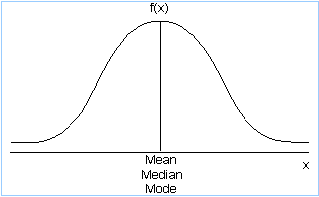### Why should I choose AnalystNotes?

AnalystNotes specializes in helping candidates pass. Period.

##### Subject 6. The Normal Distribution
Normal distributions are a family of distributions that have the same general shape.

• They are symmetrical with scores more concentrated in the middle than in the tails.
• Normal distributions are sometimes described as bell-shaped with a single peak at the exact center of the distribution.
• The tails of the normal curve extends indefinitely in both directions. That is, possible outcomes of a normal distribution lie between - ∞ and + ∞.
• Normal distributions may differ in how spread-out they are.

The graph looks like this:The key properties are:

• The normal distribution is completely described by two parameters: the mean (μ) and the standard deviation (σ).
• The normal distribution is symmetrical: it has a skewness of 0, a kurtosis (it measures the peakedness of a distribution) of 3, and an excess kurtosis (which equals kurtosis less 3) of 0. As a consequence, the mean, median, and mode are all equal for a normal random variable.
• A linear combination of two or more normal random variables is also normally distributed.

One reason the normal distribution is important is that many psychological, educational, and financial variables are distributed approximately normally. Measures of reading ability, introversion, job satisfaction, and memory are among the many psychological variables approximately normally distributed. Although the distributions are only approximately normal, they are usually quite close.

A second reason is that it is easy for mathematical statisticians to work with. Many kinds of statistical tests can be derived for normal distributions. Almost all statistical tests discussed in the textbook assume normal distributions. Fortunately, these tests work very well even if the distribution is only approximately normally distributed. Some tests work well even with very wide deviations from normality.

Finally, if the mean and standard deviation of a normal distribution are known, it is easy to convert back and forth from raw scores to percentiles.

For example, normal distribution is an approximate model for asset returns. The price of any asset can only drop to 0. Therefore, the lowest return on an asset is -100% (i.e., all investment in the asset is lost). Since the normal distribution extends to negative infinity without limit, it is not an accurate model for asset returns. However, for the normal distribution, the probability of outcomes below -100% is very small. Therefore, the normal distribution can be considered an approximate model for returns. However, the normal distribution tends to underestimate the probability of extreme returns.

Confidence intervals for a normally distributed random variable.

Analysts can use the sample mean to estimate the population mean, and the sample standard deviation to estimate the population standard deviation. The sample mean and sample standard deviation are point estimates.

Probability statements about a random variable are often framed using confidence intervals built around point estimates. In investment work, confidence intervals for a normal random variable in relation to its estimated mean are often used.

Confidence intervals use point estimates to make probability statements about the dispersion of the outcomes of a normal distribution. A confidence interval specifies the percentage of all observations that fall in a particular interval.

The exact confidence intervals for a normal random variable X:

• 90% confidence interval for X is: x-bar - 1.645σ to x-bar + 1.645σ: this means that 10% of the observations fall outside the 90% confidence interval, with 5% on each side.

• 95% confidence interval for X is: x-bar - 1.96σ to x-bar + 1.96 σ: this means that 5% of the observations fall outside the 95% confidence interval, with 2.5% on each side.

• 99% confidence interval for X is: x-bar - 2.58 σ to x-bar + 2.58 σ: this means that 1% of the observations fall outside the 99% confidence interval, with 0.5% on each side.

Hint: memorize these numbers (1.645, 1.96 and 2.58) to quickly solve relevant problems. For details about confidence intervals, refer to Reading 10 - Sampling and Estimation.

Learning Outcome Statements

j. explain the key properties of the normal distribution;

CFA® 2022 Level II Curriculum, , Volume 1, Reading 9

User Comment
achu 1.645 90%; 1.96 95% ; 2.58 99%.
momtaz The sample mean and sample standard deviation are point estimates.
anne3lance Are we expecteed to learn these figures by heart?
olagbami Yes Anne, u r to memorize dem!
Sego yeah, its just one of those things
thekobe also remember the chebysheb numbers 36% 56% and 75% 1.25 1.50 and 2
Bududeen No need to memorize chebyshev ...just know the formula 1-1/k^2
Yrazzaq88 Easy to remember, that 1.96 << 96 is close to 95% CI

Once we know that,

1.645 = lowest 90%

2.58 = highest 99%
irapp92 So does this imply that the only Z- scores that we need to know confidence intervals for are 1 (68%), 1.645 (90%), 1.96 (95%), and 2.58 (99%)? Seems pretty arbitrary... am I missing a formula somewhere? Specifically one that can provide an exact confidence variable for a given z- score?
irapp92 Just saw the final sentence "for details about confidence interval, refer to Reading - Sampling and Estimation." Analyst Notes keepin' it suspenseful with the cliffhanger!